With some instruments the gradient dwell volume can be quite significant, even a few milliliters.

At the start of the gradient, the volume in the instrument is filled with the mobile phase corresponding to the initial gradient conditions and consequently the “dwell volume” of the mobile phase should flow through the column before the starting gradient profile arrives at the top of the column. As a result the expected gradient elution is delayed and some analytes, especially weakly retained ones, may migrate a certain distance along the column during this unintended initial isocratic step, which contributes in this way to the elution volume.

This behavior can be described as a two-step elution with the first, isocratic (dwell volume) step, followed by the second, gradient, step. The dwell volume may differ from one instrument to another and may cause difficulties if a gradient HPLC method is transferred from one instrument to another one. To avoid these problems and to make possible precise prediction of the gradient elution data by calculation, the gradient dwell volume should be accounted for in method development and appropriate correction should be adopted for the instrumental gradient delay in calculations.

1. Theory

The major application of HPLC using gradient conditions is for reversed-phase (RP-HPLC) systems1, however the general conclusions are also applicable to other HPLC modes (normal-phase, Aqueous Normal Phase ANP, ion-exchange, etc.). For RP-HPLC separations, the solute retention factor k can be approximately related to the volume-fraction of organic modifier (B) in a mobile phase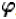as:

where kw is the (extrapolated) value of k for water as the mobile phase (=0), and S is a constant characteristic for each solute in the sample. Retention time in a linear-gradient separation can be expressed as: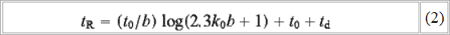Here, t0 is the column dead-time, k0 is the value of k at the start of the gradient, and b is a gradient steepness parameter given by equation 3 below: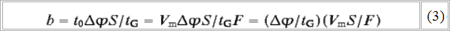whereis the change in the volume-fraction of organic modifier (B) during the gradient (=0.01%B)Vm is the column dead-volume, and F is the flow-rate. The quantity td is the equipment hold-up or ‘dwell’ time, equal to the hold-up or ‘dwell’ volume VD divided by flow-rate. The equipment dwell volume is often wrongly assumed to be negligible (VD=0), which leads to the problems. Relative retention tR/t0 can be defined, and from Eq. 2 (assuming td=0):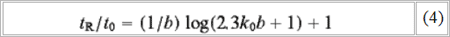If column dimensions or flow-rate are changed, t0=Vm/F will change. However, as long as Q is held constant (by varying tG), b and tR/t0 remain constant for each solute, and relative retention will not change. Eq. 2 and Eq. 4 are actually only approximations when VD>0, but it is still possible to calculate exact values of tR as a function of k0t0b and VD. Resolution in gradient elution can be expressed in terms that are similar to isocratic separation: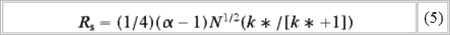where k* (the average retention factor k during gradient elution) is given as: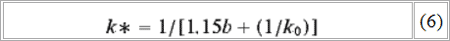For peaks that elute later in the gradient, and for which k0>1.15b,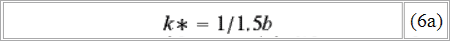2. Dwell-volume effects

According to Eq. 4 above, relative retention should remain unchanged as long as b (and Q) are constant, assumes that VD=0. When there is a significant dwell volume VD in the instrument, this situation changes. As mentioned in the opening paragraph gradient conditions are delayed by a dwell time tD=VD/F. As a result analytes is under isocratic conditions (for a time tD) with k=k0. As a result the value of b, the gradient steepness parameter (b=0 for isocratic elution) is smaller than given by 3 for the gradient elution. The composite value of b (b’; i.e. effective gradient steepness) for a separation of a sample undergoing isocratic elution at the start is therefore smaller. The effective gradient steepness b’ is therefore a combination of initial elution with b = 0 and elution with b calculated by equation 3. As a result solutes which are weakly retained (elute early) will undergo the most migration during isocratic part of the run and as a consequence for these compounds b’ will be greatest.

For later eluting compounds b’/b will approach 1 and become smaller as tR becomes smaller. This combined gradient steepness parameter b’ will depend both on k0 and the ratio of dwell volume to column volume (VD/Vm). To assure there are minimal changes in relative retention of the compounds if a gradient HPLC method is transferred from one instrument to another, one should keep both Q and (VD/Vm) constant. If VD/Vm is held constant while changing column dimensions, relative retention (tR/t0) should remain unchanged.

1 J. W. Dolan and L. R. Snyder; “Maintaining fixed band spacing when changing column dimensions in gradient elution” J. Chromatogr. A, Vol. 799, 13 March 1998, Pages 21-34.